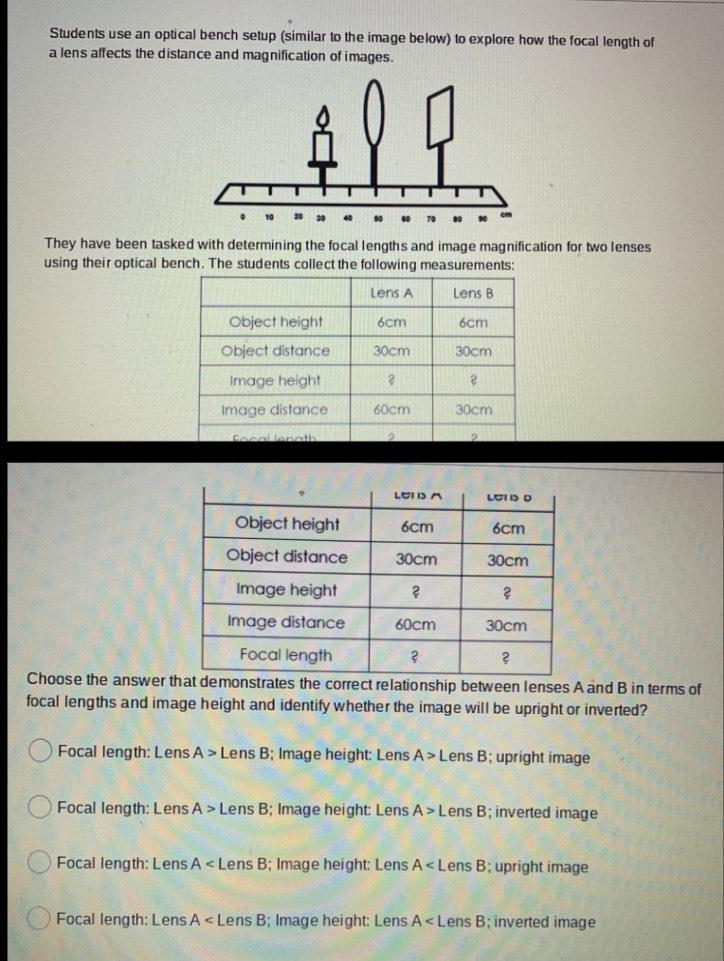Question:

# Students use an optical bench setup (similar to the image below) to explore how the focal length of a lens affects the distanceStudents use an optical bench setup (similar to the image below) to explore how the focal length of a lens affects the distance and magnification of images. & ll • 10 20 30 40 They have been tasked with determining the focal lengths and image magnification for two lenses using their optical bench. The students collect the following measurements: Lens A Lens B 6cm 6cm 30cm 30cm Object height Object distance Image height Image distance RIA 2 2 60cm 30cm LOIS LOID 6cm 6cm Object height Object distance 30cm 30cm Image height ? ? Image distance 60cm 30cm Focal length 2 ? Choose the answer that demonstrates the correct relationship between lenses A and B in terms of focal lengths and image height and identify whether the image will be upright or inverted? O Focal length: Lens A > Lens B; Image height: Lens A > Lens B; upright image Focal length: Lens A > Lens B; Image height: Lens A > Lens B; inverted image o Focal length: Lens A <Lens B: Image height: Lens A<Lens B; upright image C Focal length: Lens A < Lens B: Image height: Lens A <Lens B: inverted image